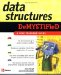# Quiz

 1 What is a tree?2 What is the relationship between parent node and child nodes?3 What are a key and a value?4 What is a root node?5 What is the purpose of a left child node and a right child node?6 What is a leaf node?7 What is the depth of a tree?8 What is the size of a tree?9 How do you calculate the size of a tree?10 Can a tree have a duplicate key?1 A tree is a data structure where data is stored in nodes. Nodes are arranged in branches where each node can expand into 0, 1, or 2 other nodes. 2 A parent node is a node that branches into one or two other nodes, which are called child nodes. 3 A key is a component of a node that identifies the node. An application searches keys to locate a desired node. A value is also a component of a node that is used to store data. 4 A root node is another term for a parent node. 5 The left child node has a key that is less than the key of its parent node. The right child node has a key that is greater than the key of its parent node. 6 A leaf node is the last node on a branch and does not have any child nodes. 7 The depth of a tree is the number of levels of a tree. 8 The size of a tree is the number of nodes on the tree. 9 You calculate the size of a tree by using the following formula: size » 2 depth If the depth is 5 levels, then the size is 32, as shown here: 32 » 2 5 10 No, a tree cannot have a duplicate key.Data Structures Demystified (Demystified)
ISBN: 0072253592
EAN: 2147483647
Year: 2006
Pages: 90

Similar book on Amazon